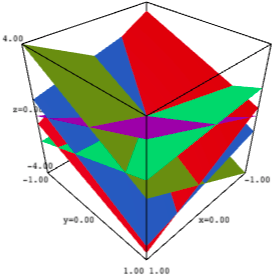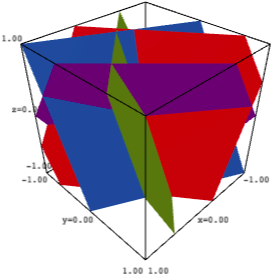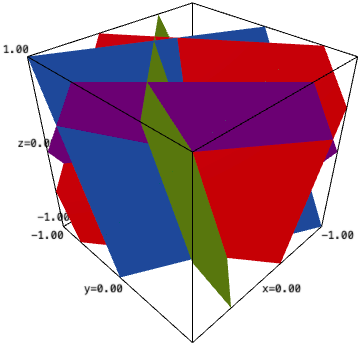# Ranging the Z axis

I am plotting arrangements of hyperplanes. Like this one:

H3.<x, y, z> = HyperplaneArrangements(QQ)
A = H3([(1, 2, 1), 0],
[(-4, -3, -2), 0],
[(3, 3, -2), 0],
[(1, -4, 3), 0],
[(-2, 2, 1), 0])
A.plot(ranges=[(-1, 1), (-1, 1)], aspect_ratio=(1, 1, 0.25))The problem is I need the z axis to range from -1 to 1 rather than from -4 to 4.

Is there a way to change that?

edit retag close merge delete

Sort by » oldest newest most voted

# Setting zmin and zmax to get a chosen z-range

## Missing functionality

Inspecting the plot method of hyperplanes arrangement reveals this is not implemented in Sage yet.

Searching Ask Sage and sage-support, we find that many 3d plotting functions similarly lack the ability to limit the z-range. Providing this missing functionality is now tracked at

## Workaround

Some of the questions on this theme were answered with a suggestion to use implicit_plot3d. For hyperplanes, this works very well.

We can therefore mimic the plot method of hyperplane arrangements and cook up a function which, if given only an x-range and a y-range, will simply call the plot method (which adapts the z-range to the full range of z values corresponding to the x-range and y-range), but if additionally given a z-range, will use implicit plots to limit the z-range.

Here is such a function:

def ha_plot(A, ranges, **opt):
r"""
Return a plot of this hyperplane arrangement.

INPUT:

- A -- a hyperplane arrangement

- ranges -- a list consisting of an x-range, a y-range,
and optionally a z-range
"""
from colorsys import hsv_to_rgb
if len(ranges) == 2:
return A.plot(ranges=ranges, **opt)
elif len(ranges) != 3:
raise ValueError("ranges should consist in two or three tuples")
hh = [h.coefficients() for h in A.hyperplanes()]
N = len(hh)
HSV_tuples = [(i*1.0/N, 0.8, 0.9) for i in range(N)]
cols = [hsv_to_rgb(*x) for x in HSV_tuples]
ff = lambda a, b, c, d: lambda x, y, z: d + a*x + b*y + c*z
ip = lambda f, col: implicit_plot3d(f, *ranges, color=col, **opt)
hp = lambda a, b, c, d, col: ip(ff(a, b, c, d), col)
return sum(hp(a, b, c, d, col) for (d, a, b, c), col in zip(hh, cols))


Usage is as follows.

One can provide only an x-range and a y-range:

sage: ha_plot(A, [(-1, 1), (-1, 1)]).show(aspect_ratio=(1, 1, 0.25))


and get the exact same picture as in the question.

Or one can additionally provide a z-range:

sage: ha_plot(A, [(-1, 1), (-1, 1), (-1, 1)]).show(aspect_ratio=1)Optional extra arguments are passed to implicit_plot3d.

This for instance allows to set the opacity:

sage: ha_plot(A, [(-1, 1), (-1, 1), (-1, 1)], opacity=0.5).show(aspect_ratio=1)


The function could be improved to accept a choice of colours. Currently it uses the same default as the plot method of hyperplane arrangements.

## Solution using the add_condition method of 3D plots

One could alternatively use the add_condition method of 3D graphics objects.

One needs to pay attention to the fact that the plot of a hyperplane arrangement is in fact the sum of the graphs of the individual hyperplanes.

The add_condition method applies to each of those, rather than to their sum.

Define the hyperplane arrangement and name its plot:

H3.<x, y, z> = HyperplaneArrangements(QQ)
A = H3([(1, 2, 1), 0],
[(-4, -3, -2), 0],
[(3, 3, -2), 0],
[(1, -4, 3), 0],
[(-2, 2, 1), 0])
a = A.plot(ranges=[(-1, 1), (-1, 1)], aspect_ratio=(1, 1, 0.25))


Define bounds and sum the reworked plots of individual arrangements truncated using add_condition:

z_bounds = lambda x, y, z: -1 < z < 1
aa = sum(h.add_condition(z_bounds) for h in a.all)


Show the result:

aamore

1

If the answer solves your problem, accept it by clicking the check mark near the top left of the answer.

This will mark the question as solved.